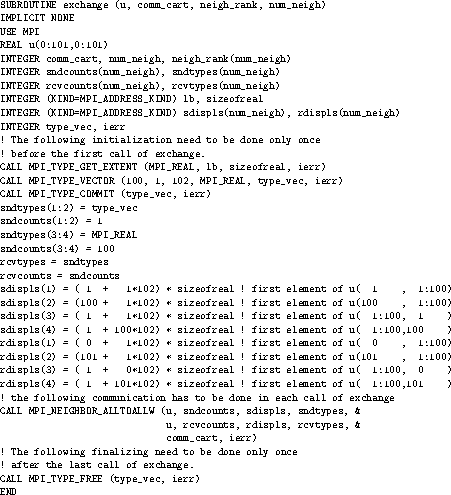# 194. An Application ExampleUp: Contents Next: MPI Environmental Management Previous: Nonblocking Neighborhood Alltoall

Example The example in Figures 18 -An Application Example shows how the grid definition and inquiry functions can be used in an application program. A partial differential equation, for instance the Poisson equation, is to be solved on a rectangular domain. First, the processes organize themselves in a two-dimensional structure. Each process then inquires about the ranks of its neighbors in the four directions (up, down, right, left). The numerical problem is solved by an iterative method, the details of which are hidden in the subroutine relax.

In each relaxation step each process computes new values for the solution grid function at the points u(1:100,1:100) owned by the process. Then the values at inter-process boundaries have to be exchanged with neighboring processes. For example, the newly calculated values in u(1,1:100) must be sent into the halo cells u(101,1:100) of the left-hand neighbor with coordinates (own_coord(1)-1,own_coord(2)).

```INTEGER ndims, num_neigh
LOGICAL reorder
PARAMETER (ndims=2, num_neigh=4, reorder=.true.)
INTEGER comm, comm_cart, dims(ndims), ierr
INTEGER neigh_rank(num_neigh), own_coords(ndims), i, j, it
LOGICAL periods(ndims)
REAL u(0:101,0:101), f(0:101,0:101)
DATA dims / ndims * 0 /
comm = MPI_COMM_WORLD
!   Set process grid size and periodicity
CALL MPI_DIMS_CREATE(comm, ndims, dims, ierr)
periods(1) = .TRUE.
periods(2) = .TRUE.
!   Create a grid structure in WORLD group and inquire about own position
CALL MPI_CART_CREATE (comm, ndims, dims, periods, reorder, &
comm_cart, ierr)
CALL MPI_CART_GET (comm_cart, ndims, dims, periods, own_coords, ierr)
i = own_coords(1)
j = own_coords(2)
! Look up the ranks for the neighbors.  Own process coordinates are (i,j).
! Neighbors are (i-1,j), (i+1,j), (i,j-1), (i,j+1) modulo (dims(1),dims(2))
CALL MPI_CART_SHIFT (comm_cart, 0,1,  neigh_rank(1),neigh_rank(2), ierr)
CALL MPI_CART_SHIFT (comm_cart, 1,1,  neigh_rank(3),neigh_rank(4), ierr)
! Initialize the grid functions and start the iteration
CALL init (u, f)
DO it=1,100
CALL relax (u, f)
!      Exchange data with neighbor processes
CALL exchange (u, comm_cart, neigh_rank, num_neigh)
END DO
CALL output (u)
```

Figure 18: Set-up of process structure for two-dimensional parallel Poisson solver.

```SUBROUTINE exchange (u, comm_cart, neigh_rank, num_neigh)
REAL u(0:101,0:101)
INTEGER comm_cart, num_neigh, neigh_rank(num_neigh)
REAL sndbuf(100,num_neigh), rcvbuf(100,num_neigh)
INTEGER ierr
sndbuf(1:100,1) = u(  1,1:100)
sndbuf(1:100,2) = u(100,1:100)
sndbuf(1:100,3) = u(1:100,  1)
sndbuf(1:100,4) = u(1:100,100)
CALL MPI_NEIGHBOR_ALLTOALL (sndbuf, 100, MPI_REAL, rcvbuf, 100, MPI_REAL, &
comm_cart, ierr)
! DO i=1,num_neigh
!   CALL MPI_IRECV(rcvbuf(1,i),100,MPI_REAL,neigh_rank(i),...,rq(2*i-1),&
!                  ierr)
!   CALL MPI_ISEND(sndbuf(1,i),100,MPI_REAL,neigh_rank(i),...,rq(2*i  ),&
!                  ierr)
! END DO
! CALL MPI_WAITALL (2*num_neigh, rq, statuses, ierr)

u(  0,1:100) = rcvbuf(1:100,1)
u(101,1:100) = rcvbuf(1:100,2)
u(1:100,  0) = rcvbuf(1:100,3)
u(1:100,101) = rcvbuf(1:100,4)
END
```

Figure 19: Communication routine with local data copying and sparse neighborhood all-to-all.Communication routine with sparse neighborhood all-to-all-w and without local data copying.Up: Contents Next: MPI Environmental Management Previous: Nonblocking Neighborhood Alltoall